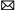info@LoadCells.net
+1 800-585-1519 (Toll-free)

Home » Wiki » What is a Load Cell

What is a Load Cell

A load cell is actually a device that converts a mass signal into a measurable electrical signal. The working principle is to convert the weight of the object added to the scale pan into an electrical signal proportional to the weight, amplify and A/D convert the output electrical signal, and then to display the weighing information by other relevant circuit.
Considering the influence of gravity acceleration and air buoyancy of different places on the conversion, the performance index of load cells mainly include linearity error, hysteresis error, repeatability error, creep, zero temperature characteristic, sensitivity temperature characteristic, etc. In various scales and mass measurement systems, the composite error is usually used to comprehensively control the load cell accuracy, and the composite error band is linked to the scale error band to select a load cell corresponding to a certain accuracy scale. The International Organization of Legal Metrology (OIML) stipulates that the error band δ of the load cell occupies 70% of the error band Δ of the scale. The sum of the linearity error, the hysteresis error, and the error caused by the influence of temperature on the sensitivity within the specified temperature range, can't exceed the error band δ.
1. Sensitive element
An element can directly sense the measured amount (mass) and output other amounts that have a defined relationship to the measurement. For example, the elastic element of strain gauge load cell converts the mass of the measured object into a deformation. The elastomer of the capacitive load cell converts the measured mass into a displacement.
2. Transform element
Transform element also known as sensing element. It converts the output of a sensitive element into a signal that is easy to measure. For example, the strain gauge of strain gauge load cell converts the deformation of the elastomer into a change of resistance. The capacitor of the capacitive load cell converts the displacement of the elastomer into a change of capacitance. Sometimes some elements have both the functions of sensitive element and transform element. For example, a piezoelectric material of a piezoelectric load cell can output electrical quantity while being deformed by an external load.
3. Measuring components
Convert the output signal of transform element into an electrical signal provides convenience for further transmission, processing, display, recording or control. Such as the bridge circuit in the resistance strain type load cell, the charge preamplifier of the piezoelectric load cell.
4. Auxiliary power supply
Auxiliary power supply provides energy for the electrical signal output of the load cell. Generally, the load cell needs an external power supply to work. Therefore, as a product, the requirements for power supply must be indicated, but not as a part of the load cell.